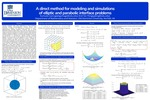## College of Sciences Posters

#### College

College of Sciences

#### Department

Mathematics and Statistics

#### Program

Computational and Applied Mathematics

4-2021

#### DOI

10.25883/bgc2-ss53

#### Abstract

Interface problems have many applications in fluid dynamics, molecular biology, electromagnetism, material science, heat distribution in engines, and hyperthermia treatment of cancer. Mathematically, interface problems commonly lead to partial differential equations (PDE) whose in- put data are discontinuous or singular across the interfaces in the solution domain. Many standard numerical methods designed for smooth solutions poorly work for interface problems as solutions of the interface problems are mostly non-smoothness or discontinuous. Moving interface problems depends on the accuracy of the gradient of the solution at the interface. Therefore, it became essential to derive a method for interface problems that gives second-order accuracy for both the solution and its gradients. As most applications in the real world are in 3D settings, the development of three-dimensional elliptic interface solvers and the study of their convergence is significant. We have developed a novel Direct Method for solving three-dimensional elliptic interface problems that preserve the discontinues in solution and gradient across the interface. The method uses a standard seven-point central difference scheme at regular grid points and a compact twenty-seven-point scheme only at irregular grid points by incorporating the interface's jump conditions. The model was implemented using both MATLAB and FORTRAN routines and showed that the computed solution and gradient of the solution near the interface are second-order accurate in infinity norm. We also have modified the developed direct method to solve the 3D heat equation with fixed interfaces. Numerical results showed that the proposed method is unconditionally stable and is second-order accurate in both time and space.

#### Keywords

Elliptic interface problem, Parabolic interface problem, Finite difference scheme, Uniform grid

#### Disciplines

Applied Mathematics | Mathematics | Partial Differential Equations

#### Files